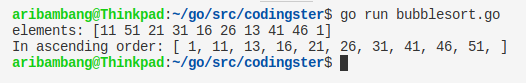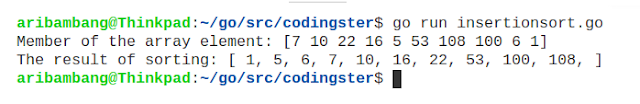-->

## Social Items

Go Program To Find Area Of Square (Golang) - This Go program to calculate an area of a square, The program takes the length of the square as input, calculates the area of the square and outputs it on the screen.

### Source Code :

``````package main

import "fmt"

func main() {
var length, area int

fmt.Print("Enter length of square: ")
fmt.Scanln(&length)

area = length * length

fmt.Println("Area of square is: ", area)
}
``````

### Compile & Run :

Here's how to compile source code manually:
``````
\$ go build areaofsquare.go
\$ ./areaofsquare
``````
Run without compile:
``````
\$ go run areaofsquare.go
``````

### Output Of Program :The result of Golang program to find area of square## Go Program To Find Area Of Square (Golang)

Go Program To Find Area Of Circle - This Go program to calculate an area of a circle, The program takes the radius of the circle as input, calculates the area of the circle and outputs it on the screen.

### Source Code :

``````package main

import "fmt"

func main() {
var radius, area float64
const phi float64 = 3.14

fmt.Print("Enter radius of circle: ")
fmt.Scanln(&radius)
area = phi * radius * radius
fmt.Println("Area of circle is: ", area)
}
``````

### Compile & Run :

Here's how to compile source code manually:
``````
\$ go build areaofcircle.go
\$ ./areaofcircle
``````
Run without compile:
``````
\$ go run areaofcircle.go
``````

### Output Of Program :The result of Golang program to find area of circle## Go Program To Find Area Of Circle (Golang)

Bubble Sort Algorithm in Go (Golang) - This code implements the bubble sort algorithm to sort numbers in ascending order. Bubble Sort is less efficient as its average and worst case complexity is high, there are many other fast sorting algorithms like quick-sort, heap-sort, etc.

### Source Code :

``````package main

import "fmt"

func bubbleSort(arr []int) {
for {
sorted := true
for i := 0; i < len(arr)-1; i++ {
if arr[i] > arr[i+1] {
tmp := arr[i]
arr[i] = arr[i+1]
arr[i+1] = tmp
sorted = false
}
}
if sorted == true {
break
}
}
}

func main() {
arr := []int{11, 51, 21, 31, 16, 26, 13, 41, 46, 1}
fmt.Print("elements: ", arr, "\n", "In ascending order: [ ")
bubbleSort(arr)
for i := 0; i < len(arr); i++ {
fmt.Print(arr[i], ", ")
}
fmt.Print("]\n")
}
``````

### Compile & Run :

Here's how to compile source code manually:
``````
\$ go build bubblesort.go
\$ ./bubblesort
``````
Run without compile:
``````
\$ go run bubblesort.go
``````

### Output Of Program :Result program bubble sort in Golang## Bubble Sort Algorithm in Go (Golang)

Selection Sort Algorithm in Go (Golang) - This code implements the selection sort algorithm to set the array of numbers in ascending order.

### Source Code :

``````package main

import "fmt"

func selectionSort(arr []int) {

for i := 0; i < len(arr)-1; i++ {
minIndex := i
for j := i + 1; j < len(arr); j++ {
if arr[minIndex] > arr[j] {
minIndex = j
}
}
tmp := arr[i]
arr[i] = arr[minIndex]
arr[minIndex] = tmp
}
}

func main() {
arr := []int{11, 8, 35, 98, 3, 44, 24, 14, 10, 1}
fmt.Print("elements: ", arr, "\n", "In ascending order: [ ")
selectionSort(arr)
for i := 0; i < len(arr); i++ {
fmt.Print(arr[i], ", ")
}
fmt.Print("]\n")
}
``````

### Compile & Run :

Here's how to compile source code manually:
``````
\$ go build selectionsort.go
\$ ./selectionsort
``````
Run without compile:
``````
\$ go run selectionsort.go
``````

### Output Of Program :Result program selection sort in Go## Selection Sort Algorithm in Go (Golang)

In this post, we will learn to create an insertion sort algorithm program in the Go programming language (Golang). We try to sort 10 numbers randomly, with numbers that will be sorted as follows: 7, 10, 22, 16, 5, 53, 108, 100, 6, 1.

### Source Code :

``````package main

import "fmt"

func insertionSort(arr []int) {

for i := 0; i < len(arr); i++ {
tmp := arr[i]
j := i
for j > 0 && arr[j-1] > tmp {
arr[j] = arr[j-1]
j--
}
arr[j] = tmp
}
}

func main() {
arr := []int{7, 10, 22, 16, 5, 53, 108, 100, 6, 1}
fmt.Print("Member of the array element: ", arr, "\n", "The result of sorting: [ ")
insertionSort(arr)
for i := 0; i < len(arr); i++ {
fmt.Print(arr[i], ", ")
}
fmt.Print("]\n")
}
``````

Save the source code with the name of insertionsort.go, don't forget the extension should .go

### Compile & Run :

Here's how to compile source code manually:
``````
\$ go build insertionsort.go
\$ ./insertionsort
``````
You can run without having to compile it:
``````
\$ go run insertionsort.go
``````

### The Output of Program :Picture of the result insertion sort algorithm## Insertion Sort Algorithm In Go (Golang)

Go Program To Check Leap Year - in this post, we will learn how to create a leap year check program in the Go programming language. Leap year is the year where the number of days is the most that occur every four years. The difference in leap year with the normal year is every February has a date up to 29.

## Go Program To Check Leap Year

### Source Code :

``````package main

import "fmt"

func main() {
var year int

fmt.Print("Enter a year: ")
fmt.Scanln(&year)

if year%400 == 0 {
fmt.Println(year, "is a leap year")
} else if year%100 == 0 {
fmt.Println(year, "is not a leap year")
} else if year%4 == 0 {
fmt.Println(year, "is a leap year")
} else {
fmt.Println(year, "is not a leap year")
}
}
``````

Save the source code with the name of leapyear.go, but adjust wrote with a file name that chills and don't forget the extension should .go

### Compile & Run :

Here's how to compile source code manually:
``````
\$ go build leapyear.go
\$ ./leapyear
``````
You can run without having to compile it:
``````
\$ go run leapyear.go
``````

### The Output of Program :Picture of the result check leap year## Go Program To Check Leap Year (Golang)

Go Program To Generate Fibonacci Series (Golang) - in this post we will learn how to create a Fibonacci series program in the Go programming language. Fibonacci is a pattern of numbers obtained from the sum of the two previous numbers in a sequence.

## Go Program To Generate Fibonacci Series (Golang)

### Source Code :

``````package main

import "fmt"

func fibonacci(n int) int {
if n == 0 || n == 1 {
return n
} else {
return (fibonacci(n-1) + fibonacci(n-2))
}
}

func main() {
var n, i, j int
j = 0

fmt.Print("Enter the number of terms: ")
fmt.Scanln(&n)

fmt.Print("Fibonacci series: ")
for i = 1; i <= n; i++ {
fmt.Print(fibonacci(j), ", ")
j++
}
fmt.Println()
}
``````

Save the source code with the name of fibonacci.go, but adjust wrote with a file name that chills and don't forget the extension should .go

### Compile & Run :

Here's how to compile source code manually:
``````
\$ go build fibonacci.go
\$ ./fibonacci
``````
You can run without having to compile it:
``````
\$ go run fibonacci.go
``````

### The Output of Program :Picture of the result Fibonacci series## Go Program To Generate Fibonacci Series (Golang)

Go Program To Find Transpose Of A Matrix (Golang) - In this post, we will learn how to create to find transpose of a matrix program in the Go programming language. Matrix is a collection of numbers arranged in rows (vertical) and columns (horizontal) can also be called two-dimensional arrays. Matrix Transpose produces a matrix by exchanging rows into columns and columns into rows of a matrix.

## Go Program To Find Transpose Of A Matrix (Golang)

### Source Code :

``````package main

import "fmt"

func main() {
var i, j, m, n int
var matrix int
var transpose int

fmt.Print("Enter the number of rows the matrix: ")
fmt.Scanln(&m)
fmt.Print("Enter the number of columns th matrix: ")
fmt.Scanln(&n)

fmt.Println("Enter the matrix elements")
for i = 0; i < m; i++ {
for j = 0; j < n; j++ {
fmt.Scan(&matrix[i][j])
}
}

for i = 0; i < m; i++ {
for j = 0; j < n; j++ {
transpose[j][i] = matrix[i][j]
}
}

fmt.Println("Transpose of matrix: ")
for i = 0; i < n; i++ {
for j = 0; j < m; j++ {
fmt.Print(transpose[i][j], "\t")
}
fmt.Println()
}
}
``````

Save the source code with the name of transpose.go, but adjust wrote with a file name that chills and don't forget the extension should .go

### Compile & Run :

Here's how to compile source code manually:
``````
\$ go build transpose.go
\$ ./transpose
``````
You can run without having to compile it:
``````
\$ go run transpose.go
``````

### The Output of Program :Picture of the result transpose matrix

### Conclusion :

From the results of the program can be run without any error and display the results matrix transpose inputted by the user.## Go Program To Find Transpose Of A Matrix (Golang)

Subscribe Our Newsletter